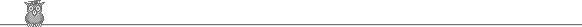### 17th Putnam 1957Problem B5

Let S be a set and P the set of all subsets of S. f : P → P is such that if X ⊆ Y, then f(X) ⊆ f(Y). Show that for some K, f(K) = K.

Solution

Let K be the union of all subsets A of S such that A ⊆ f(A). We show that K ⊆ f(K). Take any x in K. Then x belongs to some A which satisfies A ⊆ f(A). But A ⊆ K, so f(A) ⊆ f(K). So x belongs to A ⊆ f(A) ⊆ f(K), so x belongs to f(K).

Since K ⊆ f(K), we have f(K) ⊆ f( f(K) ). Hence by definition f(K) ⊆ K.